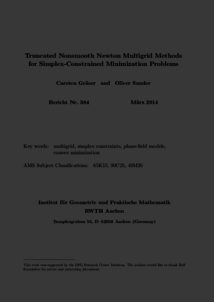Repository: Freie Universität Berlin, Math Department

# Truncated nonsmooth Newton multigrid methods for simplex-constrained minimization problems

Gräser, C. and Sander, O. (2014) Truncated nonsmooth Newton multigrid methods for simplex-constrained minimization problems. Preprint 384, IGPM Aachen . (Submitted)Preview

954kB

Official URL: https://www.rwth-aachen.de/go/id/doec/file/230298

## Abstract

We present a multigrid method for the minimization of strongly convex functionals defined on a finite product of simplices. Such problems result, for example, from the discretization of multi-component phase-field problems. Our algorithm is globally convergent, requires no regularization parameters, and achieves multigrid convergence rates. We present numerical results for the vector-valued Allen--Cahn equation and observe that the convergence rate is independent from the temperature parameter and the number of components.

Item Type: Article Mathematical and Computer Sciences > Mathematics > Numerical Analysis Department of Mathematics and Computer Science > Institute of Mathematics 1823 Ekaterina Engel 06 Mar 2016 12:29 03 Mar 2017 14:42

Repository Staff Only: item control page